# Convective Heat Transfer: One Dimensional (Part - 2) Chemical Engineering Notes | EduRev

## Chemical Engineering : Convective Heat Transfer: One Dimensional (Part - 2) Chemical Engineering Notes | EduRev

The document Convective Heat Transfer: One Dimensional (Part - 2) Chemical Engineering Notes | EduRev is a part of the Chemical Engineering Course Heat Transfer for Engg..
All you need of Chemical Engineering at this link: Chemical Engineering

3.2 Individual and overall heat transfer coefficient
If two fluids are separated by a thermally conductive wall, the heat transfer from one fluid to another fluid is of great importance in chemical engineering process plant. For such a case the rate of heat transfer is done by considering an overall heat transfer coefficient. However, the overall heat transfer coefficient depends upon so many variables that it is necessary to divide it into individual heat transfer coefficients. The reason for this becomes apparent if the above situation can be elaborated as discussed in the following sub-sections.

3.2.1 Heat transfer between fluids separated by a flat solid wall
As shown in fig.3.2, a hot fluid is separated by solid wall from a cold fluid. The thickness of the solid wall is l, the temperature of the bulk of the fluids on hot and cold sides are Th and Tc, respectively. The average temperature of the bulk fluid is T1 and T4, for hot and cold fluid, respectively. The thicknesses of the fictitious thin films on the hot and cold sides of the flat solid are shown by δ1 and δ2. It may be assumed that the Reynolds numbers of both the fluids are sufficiently large to ensure turbulent flow and the surfaces of the solid wall are clean.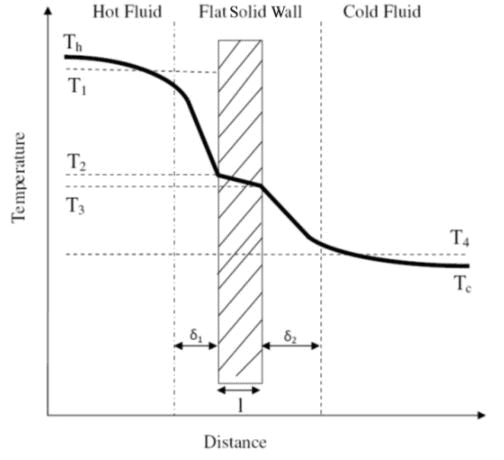Fig.3.2. Real temperature profile

It can be seen that the temperature gradient is large near the wall (through the viscous sublayer), small in the turbulent core, and changes rapidly in the buffer zone (area near the interface of sublayer and bulk fluid). The reason was discussed earlier that the heat must flow through the viscous sublayer by conduction, thus a steep temperature gradient exists because of the low temperature gradient of most of the fluids.

The average temperatures of the warm bulk fluid and cold bulk fluids are slightly less than the maximum temperature Th (bulk temperature of hot fluid) and slightly more than the minimum temperature Tc (bulk temperature of cold fluid), respectively. The average temperatures are shown by T1 and T4, for the hot and cold fluid streams, respectively.

Figure 3.3 shows the simplified diagram of the above case, where T2 and T3 are the temperatures of the fluid wall interface.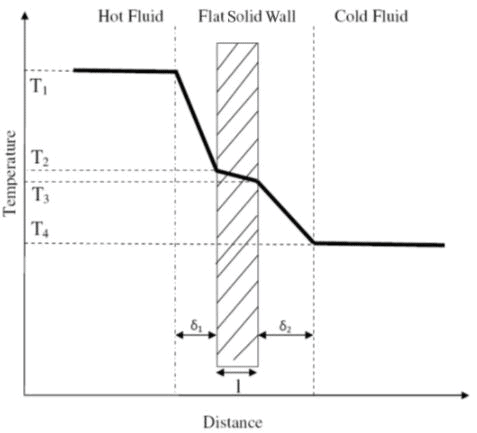Fig.3.3. Simplified temperature profile for fig.3.2

If the thermal conductivity of the wall is k, and the area of the heat transfer is A, the electrical analogy of the fig.3.3 can be represented by fig.3.4, where h1 and h2 are the individual heat transfer coefficient of the hot and cold side of the fluid.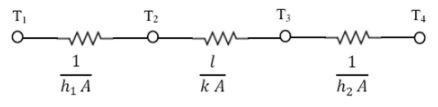Fig.3.4. Equivalent electrical circuit for fig. 3.3

Considering that the heat transfer is taking place at the steady-state through a constant area and the heat loss from other faces are negligible, then the rate of heat transfer on two sides of the wall will be represented by eq. 3.4-3.6.

Rate of heat transfer from the hot fluid to the wall,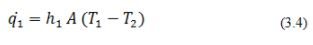Rate of heat transfer through the wall,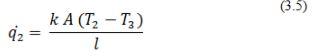Rate of heat transfer from the wall to cold fluid,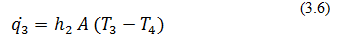At steady state, the rate of heat transfers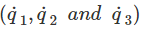are same and can be represented by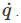Therefore,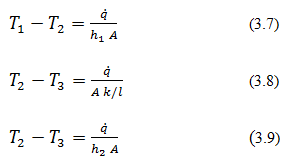On adding equations (3.7 to 3.9)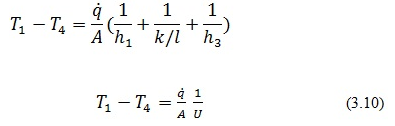where,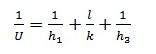Thus,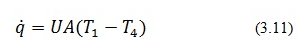The quantity U is called the overall heat transfer coefficient (can be calculated if the h1, h2, k, and l are known). Thus from the system described is established that the overall heat transfer coefficient is the function of individual heat transfer coefficient of the fluids on the two sides of the wall, as well as the thermal conductivity of the flat wall. The overall heat transfer coefficient can be used to introduce the controlling term concept. The controlling resistance is a term which possesses much larger thermal resistance compared to the sum of the other resistances. At this point it may be noted that in general the resistance offered by the solid wall is much lower. Similarly, if a liquid and a gas are separated by a solid wall the resistance offered by the gas film may generally be high.

Illustration 3.1.

The steady state temperature distribution in a wall is , where x (in meter) is the position in the wall and T is the temperature (in oC). The thickness of the wall is 0.2 m and the thermal conductivity of the wall is 1.2 (W/m·oC). The wall dissipates the heat to the ambient at 30 oC. Calculate the heat transfer coefficient at the surface of the wall at 0.2 m.

Solution 3.1
The rate of heat transfer through the wall by conduction will be equal to the rate of heat transfer from the surface to the ambient by convention at steady state,

Rate of heat transfer by conduction at x=0.2 is given by,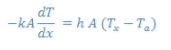where Ta is the ambient temperature.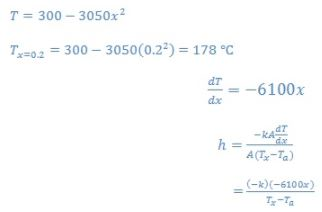On putting the values and solving,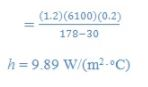Offer running on EduRev: Apply code STAYHOME200 to get INR 200 off on our premium plan EduRev Infinity!

## Heat Transfer for Engg.

46 videos|60 docs|59 tests

,

,

,

,

,

,

,

,

,

,

,

,

,

,

,

,

,

,

,

,

,

;